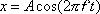For an object that is undergoing simple harmonic motion, this equation gives the position x of an object as a function of time t, for the case in which, at time zero, the object is at rest at its maximum positive displacement from the equilibrium position.

x is the position of the object with respect to its equilibrium position.
A is the amplitude of oscillations.
f is the frequency of oscillations
t is time

 Pitfall Avoidance Notes: 1. The t for time is a lower case t.  It is a variable.  Don't confuse it with the period of oscillations T.  The period is represented by an upper case T.   The period T is a constant. 2. People often confuse the position x with the amplitude A. For an object in simple harmonic motion, the amplitude A is a constant. It is the maximum distance that the object ever finds itself from its equilibrium position. x on the other hand is a variable. It depends on the time t. It is the position of the object at time t. x varies as the object oscillates, but it is always somewhere from -A to A (inclusive).